### Objectives

1. To know the definition of a projectile and to use concepts of force and inertia to explain the manner in which gravity affects a projectile.
2. To be able to describe the horizontal and vertical components of the velocity of a projectile.
3. To be able to describe the horizontal and vertical components of the displacement of a projectile.
4. To be able to numerically describe the various features associated with a projectile’s trajectory (e.g., components
5. To use kinematic equations to analyze and solve horizontally-launched projectile problems.
6. To use kinematic equations to analyze and solve angle-launched projectile problems.

### Readings from The Physics Classroom Tutorial

Motion and Forces in Two Dimensions Chapter, Lesson 2

### Interactive Simulations

1. Projectile Simulator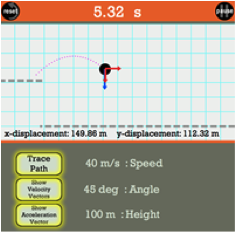This projectile simulator allows students to alter the launch speed, launch height and launch angle of a projectile. The trajectory, range, and time of fight are displayed. The velocity vector components, acceleration vector, and the trajectory trace can be toggled off and on. The simulator is accompanied by three separate exercises that emphasize various aspects of projectile motion. The simulation is an HTML5 simulation that was designed with iPads, Chromebooks, and smart phones as the target device.

2. PhET: Projectile Motion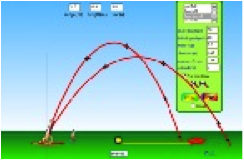In this simulation, students can fire various objects out of a cannon, including a golf ball, football, pumpkin, human being, a piano, and a car.  By manipulating angle, initial speed, mass, and air resistance, concepts of projectile motion come to light in a fun and game-like environment. Can you set the initial conditions so that you hit the target?  Teachers: For a unique activity to accompany this simulation, here’s a Problem-Based Learning exercise developed by a high school teacher. Learners take the role of a military artillery officer with the task of explaining factors that affect the path of a projectile fired from a cannon. The lesson is aimed at beginners as they explore variables affecting trajectory.

Projectile Motion Simulation: Problem-Based Learning  Investigation

3. Projectile Motion with Angry BirdsThis resource uses the free Tracker Video Analysis tool to measure and analyze the motion of an angry bird projected from a slingshot to hit a pig.  If you don’t have Tracker yet, here’s a link to the free download: Download Tracker Video Analysis and Modeling Tool

4. Projectile Motion: Galileo and Newton Simulation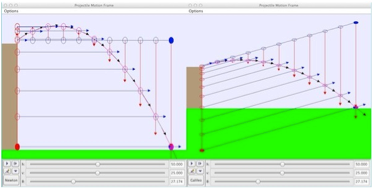In the mood to try something different? This unique resource models two views of projectile motion. The Galileo version describes projectile motion as a combination of horizontal motion with a constant velocity, equal to the projectile initial horizontal velocity component, and vertical motion with constant acceleration and an initial velocity equal to the projectile's initial velocity component. The second view, described by Newton in his Principia presents projectile motion as a combination of inertial motion, with constant velocity equal to the projectile's initial velocity and motion produced by a constant downward acceleration starting from rest. These two views are mathematically equivalent, but each emphasizes different aspects of the motion.

### Video and Animations

1. Physlet Physics: Projectile Motion Illustration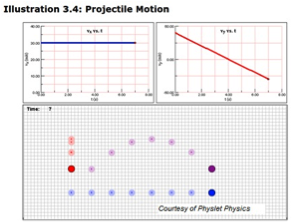This animation was designed to help beginners form correct conceptual understanding of projectile motion. It will help students visualize an object's motion in the x and y directions separately, which is key to solving projectile motion problems. Authored by Aaron Titus, a well-known and respected developer of simulations for physics education.

2. Physlet Physics: Uphill and Downhill Projectile Motion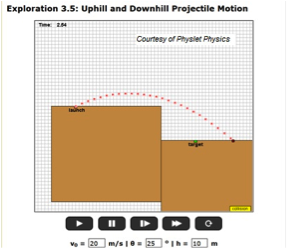This simulation with accompanying problem set would be ideal for a digital lab on projectile motion. The worksheet was crafted to help learners stay focused on specific questions limited in scope to launch angle and initial speed. It's a solid way to introduce learners to projectile motion without overwhelming them with too many variables.

3. Physlet Physics: The Projectile and the LamborghiniHere's a fun, short activity that presents a projectile aimed at a moving car. You can change the projectile's launch angle and initial speed by using the text boxes and clicking "set values and play." The task: find the relationship between v0 and θ such that the projectile will always hit the car.

### Labs and Investigations

1. The Physics Classroom, The Laboratory, Basketball Analysis

Students use video analysis to investigate the horizontal and vertical velocity and acceleration of a basketball.

2. The Physics Classroom, The Laboratory, Projectile Simulation

Students use an online simulation to investigate the motion parameters of a projectile at a variety of locations along its trajectory.

3. The Physics Classroom, The Laboratory, Projectile Problem-Solving

Students use an online application to master three types of horizontally-launched projectile problems. Students input answers and receive immediate feedback.

4. The Physics Classroom, The Laboratory, Launcher Speed

Students fire a projectile launcher horizontally from a table top and make measurements in order to determine the launch speed of the projectile launcher.

5. The Physics Classroom, The Laboratory, Maximum Range

Students use a projectile launcher to experimentally determine which angle projects a launched ball the furthest.

6. The Physics Classroom, The Laboratory, Hit the Target

Students use a calibrated projectile launcher (from Lab 4: Launcher Speed above) and predict the initial height a target a known distance away must have in order for the launched projectile to strike the target.

### Demonstration Ideas

1. MIT Tech TV: Monkey and a GunIt’s the classic scenario of "Monkey and the Hunter". A stuffed monkey is suspended from a rod. A golf-ball gun is aimed directly at the monkey. The demo is designed so the monkey begins falling precisely when the gun fires the golf ball. The projectile and target meet in mid air. Teachers: Intuitively, your students may think that the hunter needs to aim below the monkey due to the high speed of the projectile. They will reason, "If you shoot at the monkey, won’t the bullet whiz over its head as the monkey falls? But that's wrong. Once the bullet exits the gun, there’s only one force acting on it with any significance: gravity (and some negligible air resistance in this case). Likewise, only gravity will act upon the monkey after he loosens his grasp."

2. Ball Drop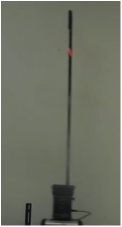This 90-second video from The Physics Classroom's YouTube Channel demonstrates and explains why a ball launched upward from a platform moving at a constant speed will fall back onto the platform. This demonstration is based on a classic question in physics: if a heavy package is released from the cargo pit of a plane that is flying at a constant speed, where will the package land when it hits the ground – under the plane, behind the plane, or in front of the plane? If the equipment is not available to perform the demonstration, then this video would be an effective alternative.

3. Upward LaunchThis 90-second video from The Physics Classroom's YouTube Channel demonstrates and explains why a ball released from above a platform moving at a constant speed will land onto the platform. The demonstration is based on a classic question in physics: If a ball is projected vertically from the bed of a pick-up truck moving at a constant speed, where will the ball land when it falls back to the ground? If the equipment is not available to perform the demonstration, this video would be an effective alternative.

### Minds On Physics Internet Modules:

The Minds On Physics Internet Modules are a collection of interactive questioning modules that target a student’s conceptual understanding. Each question is accompanied by detailed help that addresses the various components of the question.
1. Vectors and Projectiles, Ass’t VP7 -  The Nature of a Projectile
2. Vectors and Projectiles, Ass’t VP8 -  The Acceleration and Velocity of a Projectile
3. Vectors and Projectiles, Ass’t VP9 -  Velocity Components for a Projectile
4. Vectors and Projectiles, Ass’t VP10 -  Displacement and Time

### Concept Building Exercises:

1. The Curriculum Corner, Vectors and Projectiles, Projectile Motion

### Problem-Solving Exercises:

1. The Calculator Pad, Vectors and Projectiles, Problems #21 - #34

### Science Reasoning Activities:

1. Science Reasoning Center, Vectors and Projectiles, Up and Down
2. Science Reasoning Center, Vectors and Projectiles, Maximum Range of a Projectile
3. Science Reasoning Center, Vectors and Projectiles, Juggling

### Interactive Homework Problems

1. Illinois PER Interactive Example: Projectile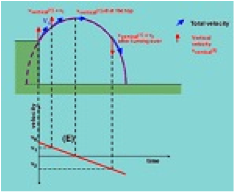This problem requires the student to determine the distance a projectile will travel when thrown off a cliff with a given initial velocity. It provides explicit help in differentiating the vertical and horizontal components of motion, then determining the correct application of formulas. The problem is accompanied by a sequence of questions designed to encourage critical thinking and conceptual analysis before dealing with the mathematics.

2. Illinois PER Interactive Example:  Arrow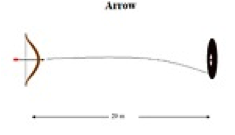This interactive problem will help learners understand why we deal with kinematic equations for both components of motion in the example of an arrow shot from a bow. Given initial velocity and distance traveled by the arrow, how much time does it take to reach the target? The author provides a detailed conceptual analysis of how to examine the horizontal component of motion (caused by the archer) and the vertical component (caused by gravity). To keep things simple, the problem assumes no air resistance.

3. Illinois PER Interactive Examples:   Thrown Ball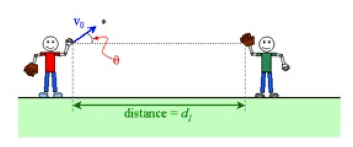Two boys, at a distance 63 meters apart, are playing catch. Given the initial speed and angle of projection of the thrown ball, how far must the catcher move to intercept the ball? A user-activated "help" sequence is provided for each step of the problem-solving, from conceptual analysis through quantitative calculation. Immediate feedback is received for both correct and incorrect responses.

### Real Life Connections:

1. Problem-Based Learning:  Murder You Solve Group Learning Activity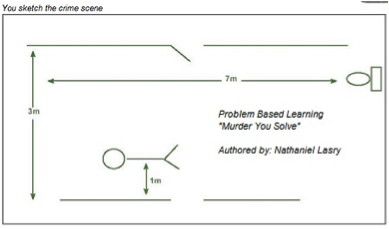Students take the role of a crime scene investigator as they explore firearm ballistics to solve a homicide. The scenario involves the case of a young man slain in his own apartment with a handgun. Students must use the ballistic data available to determine whi ch suspect is the shooter. True to the PBL method, learners will separate useful from irrelevant data, then collaborate to apply physics to solve the case. Includes a printable student manual. The Instructor's Guide (with teaching tips and solutions) requires a password, accessible at no cost to registered teacher-users.

### Common Misconceptions:

1. Horizontal Launches vs. Vertical Drops from the Same Height

A common question that quickly uncovers a misconception is "If a ball is released from rest at the same time and from the same height that a second ball is launched horizontally, then which ball will strike the ground first." Quite surprising to students, the answer is that the balls strike the ground at the same time. Imparting an initial horizontal velocity to the second ball has no affect on its vertical motion. Perpendicular components of motion are independent of each other. The misconception uncovered by the leading question is that the two components of motion somehow depend upon one another. In students' minds, the changing of a horizontal parameter affects the vertical motion.

2. Horizontal Velocity Decreases with Time

By definition, a projectile is an object upon which the only force is gravity. Gravity, being a vertical force, can only affect the vertical motion of a projectile. As such, the horizontal motion obtained at launch time does not change over the course of the motion. In the absence of horizontal forces, there is no horizontal acceleration for a projectile. The presence of air resistance would cause a launched object to decrease its horizontal velocity; but introducing air resistance into the discussion changes the topic from projectile motion to non-projectile motion.

### Elsewhere on the Web:

1. Dot Physics: Could the Black Arrow Actually Kill a Dragon?If you and your students liked The Desolation of Smaug, you'll be delighted with this exploration by physics teacher Rhett Allain. He asks the question, "Could the black arrow actually kill a dragon?" Then he takes a deep dive into the physics of the situation. He took a video clip from the trailer that shows a dwarf shooting a Black Arrow. "It shows just enough frames with the arrow in it for me to get a plot of the position."  Dr. Allain was able to plot both the horizontal and vertical position of the end of the arrow as it is shot from the ballista. He uses data from this graph, plus his own experimental data, to make a claim. Do you agree with his conclusion?

### Standards:

A. Next Generation Science Standards (NGSS)

Disciplinary Core Ideas
• HS-PS2.1.i  Newton’s second law accurately predicts changes in the motion of macroscopic objects

Crosscutting Concepts

Scale, Proportion, and Quantity
• Algebraic thinking is used to examine scientific data and predict the effect of a change in one variable on another

Systems and System Models
• When investigating or describing a system, the boundaries and initial conditions of the system need to be defined.

Science and Engineering Practices

Practice #1: Analyzing and Interpreting Data
• Analyze data using computational models in order to make valid and reliable scientific claims.

Practice #2: Developing and Using Models
• Develop and use a model based on evidence to illustrate the relationships between systems or between components of a system.
• Use a model to provide mechanistic accounts of phenomena.

Practice #3: Planning and Carrying Out Investigations
• Plan and conduct an investigation individually and collaboratively to produce data to serve as the basis for evidence … and consider limitations on the precision of the data
• Select appropriate tools to collect, record, analyze, and evaluate data.
• Collect data about a complex model or system to identify failure points or improve performance relative to criteria for success or other variables.

Practice #6: Constructing Explanations
• Construct and revise an explanation based on valid and reliable evidence obtained from a variety of sources (including students’ own investigations, models, theories, simulations) and the assumption that theories and laws that describe the natural world operate today as they did in the past and will continue to do so in the future.

Practice #8: Obtaining, Evaluating, and Communicating Information: High School
• Use mathematical representations of phenomena to support claims.
• Use mathematical representations of phenomena to describe explanations.
Create or revise a computational model or simulation of a phenomenon, designed device, process, or system.

B. Common Core Standards for Mathematics – Grades 9-12

Standards for Mathematical Practice:
• Reason abstractly and quantitatively
• Model with mathematics
• Look for and express regularity in repeated reasoning

Number and Quantity – Vector and Matrix Quantities
• N-VM.1     Recognize vector quantities as having both magnitude and direction. Represent vector quantities by directed line segments and use appropriate symbols for vectors and their magnitudes.
• N-VM.2     Find the components of a vector.

Algebra – Reasoning with Equations and Inequalities
• A-REI.4.b     Solve quadratic equations by inspection (e.g., for x squared = 49), taking square roots, completing the square, the quadratic formula, and factoring, as appropriate to the initial form of the equation.
• A-REI.10     Understand that the graph of an equation in two variables is the set of all its solutions plotted in the coordinate plane, often forming a curve.

Functions – Interpreting Functions
• F-IF.4     For a function that models a relationship between two quantities, interpret key features of graphs and tables in terms of the quantities, and sketch graphs showing key features given a verbal description of the relationship.
• F-IF.6     Calculate and interpret the average rate of change of a function (presented symbolically or as a table) over a specified interval. Estimate the rate of change from a graph.

Functions – Trigonometric Functions
• Use special triangles to determine geometrically the values of sine, cosine, tangent.

C. Common Core Standards for English/Language Arts (ELA) – Grades 9-12

Reading Standards for Literacy in Science and Technical Subjects

Key Ideas and Details: High School
• RST.9-10.2    Determine the central ideas or conclusions of a text; trace the text’s explanation or depiction of a complex process, phenomenon, or concept; provide an accurate summary of the text.
• RST.9-10.3    Follow precisely a complex multistep procedure when carrying out experiments, taking measurements, or performing technical tasks, attending to special cases or exceptions defined in the text.

Craft and Structure – High School
• RST.11-12.4     Determine the meaning of symbols, key terms, and other domain-specific words and phrases as they are used in a specific scientific or technical context relevant to grades 11-12 texts and topics.

Integration of Knowledge and Ideas – High School
• RST.11-12.7     Integrate and evaluate multiple sources of information presented in diverse formats and media in order to address a question or solve a problem.
• RST.11-12.8     Evaluate the hypotheses, data, analysis, and conclusions in a science or technical text, verifying the data when possible and corroborating or challenging conclusions with other sources of information.
• RST.11-12.9      Synthesize information from a range of sources (e.g., texts, experiments, simulations) into a coherent understanding of a process, phenomenon, or concept, resolving conflicting information when possible.)

Range of Reading and Level of Text Complexity – High School
• RST-9-10.10     By the end of Grade 10, read and comprehend science/technical texts in the grades 9-10 text complexity band independently and proficiently.